In previous article, we mentioned how to Read Values from appsettings.json in .NET Core but in this article, I have mentioned what is difference between foreach() and parallel.forach() or you can say I have explained Foreach() vs Parallel.Foreach() with an example.

`Foreach()` and `Parallel.Foreach()` both are used to loop through each item of a list, the only difference between both of them is that `Foreach()` uses single thread to loop list items, while `parallel.foreach()` can use multiple threads at same time, thus it makes parallel.foreach() loop faster when there are lots of items in list.

Let's understand it by considering a example. Let’s say we have list of string contained with names. The main goal is finding the name "Steve" as shown in below C# Code.

``````       static string[] names = new string[] { "John", ",Jakson", "Arthur", "Steve" };
static void Main(string[] args)
{
foreach (var item in names)
{
if (item == "Steve")
{
Console.WriteLine("Found the guy!");
}
}

}``````

So, as you can see in the above code, we have used a simple loop itteration by using foreach loop.

According to the goal, we have just add a condition with "Steve" string and just write a success message to the console.

We need to know the completion time to compare later, so we can add a timer to find out.

``````            Console.WriteLine("Names count: "+ names.Length);
var stopwatch = Stopwatch.StartNew(); // add this line
foreach (var item in names)
{
if (item == "Steve")
{
Console.WriteLine("Found the guy!");
Console.WriteLine(stopWatch.Elapsed.TotalSeconds.ToString()); // and this one.
}
}``````

Now, we check the output time consumed for the above loop would be as below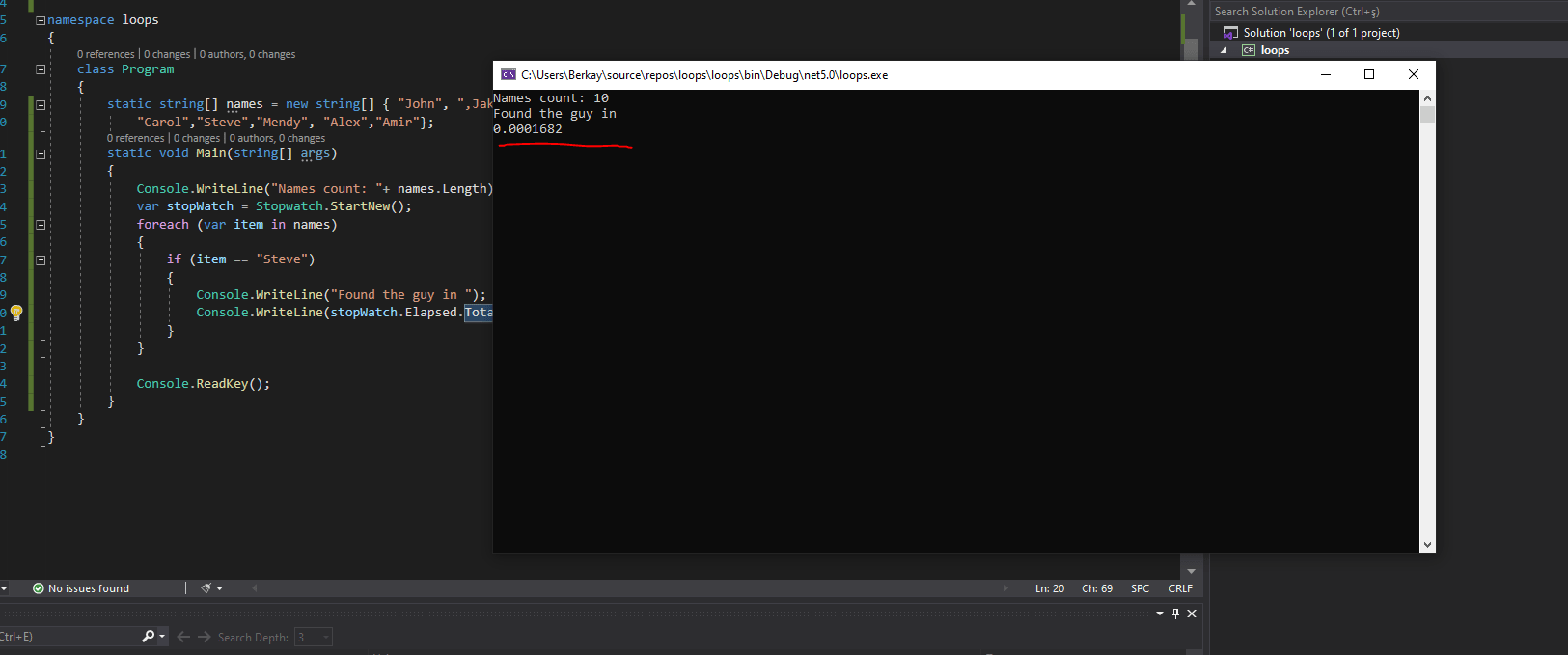What If we have much more names. Will this code run fast as above? Let's consider below code as we will add more names and check output

``````using System;
using System.Diagnostics;

namespace ForeachParallel
{
internal class Program
{

static string[] names = new string[] { "John", ",Jakson", "Arthur", "Dan","Raj",
"Carol","Steve","Mendy", "Alex","Amir", "Ali","Fatma",
"Cale","Cane", "Zayn","Faust", "Goethe", "Pep","Sydney",
"Ray" ,"Wayne","Clay", "Thomas", "Thompson","Freya", "Ragnar",
"Haaland", "Cris", "Robert", "Angela", "Micheal" ,"Pam",
"Jim", "Trent", "Max", "Lewis"};

static void Main(string[] args)
{
Console.WriteLine("Names count: "+ names.Length);
var stopwatch = Stopwatch.StartNew();

foreach (var item in names)
{
if (item == "Steve")
{
Console.WriteLine("Found the guy!");
Console.WriteLine(stopWatch.Elapsed.TotalSeconds.ToString());
}
}

}

}
}
``````

and here is the output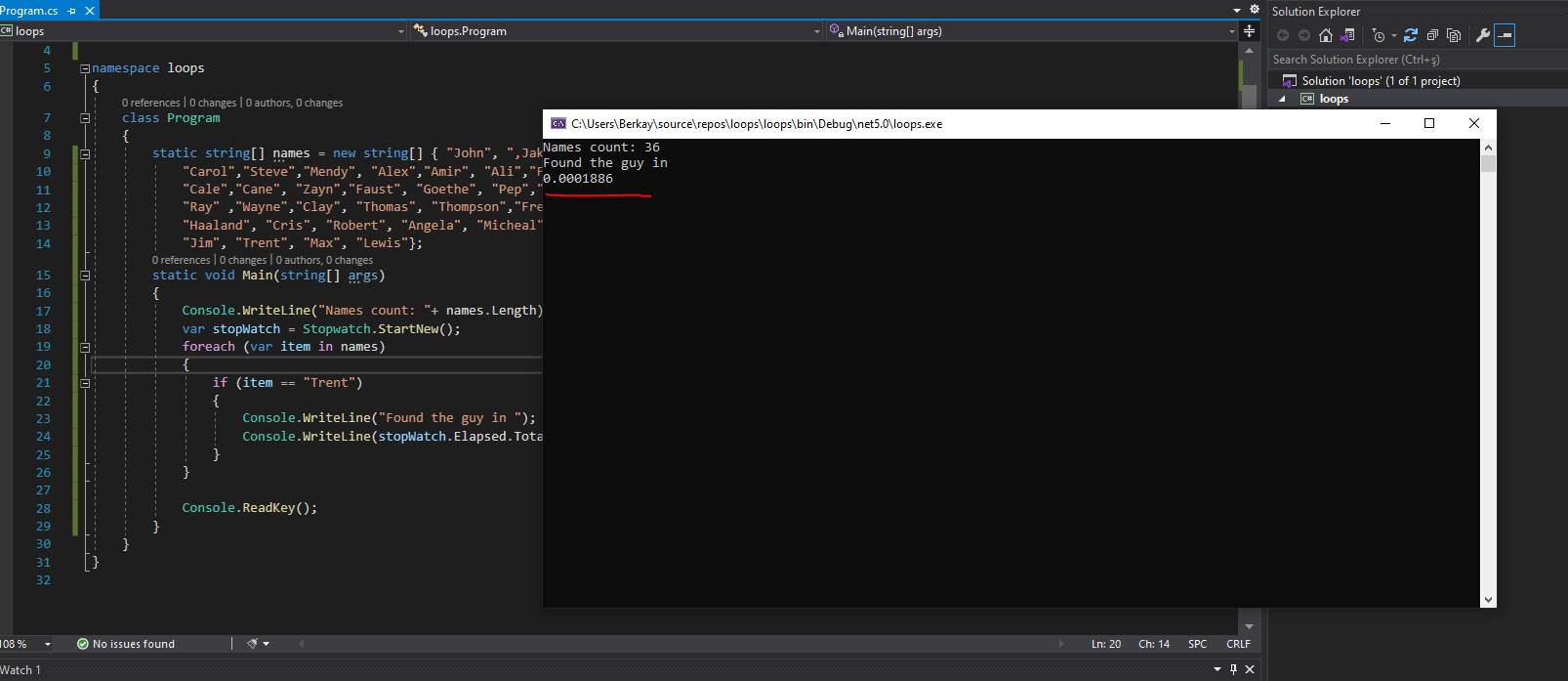As you can see, when the amount of items in array increase, elapsed of time will be increased too! Because traditional foreach runs on a single thread.

There is another thing we can try to make this iteration faster,, Which is called "Parallel foreach". Paralel foreach is basically doing same thing with traditional foreach.

It only seperates the operation between threads to complete operation faster. If we don't give ParallelOptions, it will use all available thread as much as it needs.

If we create `ParallelOptions` with `MaxDegreeOfParallelism` it will create threads the value of `MaxDegreeOfParallelism`.

Let's try adding to loop same list using Parallel.Foreach() also, as shown in below code

``````using System;
using System.Diagnostics;

namespace ForeachParallel
{
internal class Program
{
static string[] names = new string[] { "John", ",Jakson", "Arthur", "Dan","Raj",
"Carol","Steve","Mendy", "Alex","Amir", "Ali","Fatma",
"Cale","Cane", "Zayn","Faust", "Goethe", "Pep","Sydney",
"Ray" ,"Wayne","Clay", "Thomas", "Thompson","Freya", "Ragnar",
"Haaland", "Cris", "Robert", "Angela", "Micheal" ,"Pam",
"Jim", "Trent", "Max", "Lewis"};
static void Main(string[] args)
{
Console.WriteLine("Names count: " + names.Length);
var stopWatch = Stopwatch.StartNew();
foreach (var item in names)
{
if (item == "Steve")
{
Console.WriteLine("Found the guy in ");
Console.WriteLine(stopWatch.Elapsed.TotalSeconds.ToString());
}
}

stopWatch = Stopwatch.StartNew();
Parallel.ForEach(names, name =>
{
if (name == "Steve")
{
Console.WriteLine("Found the guy in ");
Console.WriteLine(stopWatch.Elapsed.TotalSeconds.ToString());
}
});
}

}
}
``````

Output: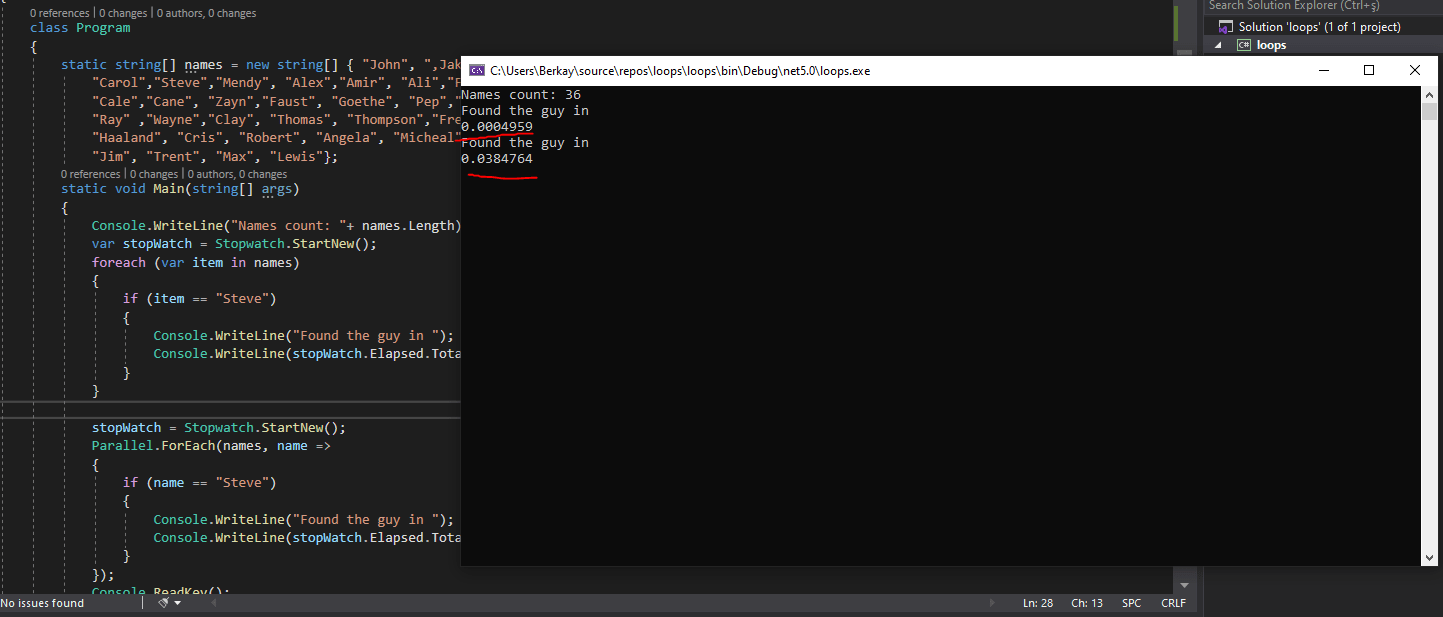As you can see in above output parallel.foreach() is taking more time than foreach(), reason for that is, there aren't lots of list items, so if list items are less we can use traditional foreach()

Iterating over simple tasks not requires any parallelism. It will make performance much worse. When tasks are not simple like this, then we can rely on Parallel Foreach! Let’s make some complex itterations.

Let’s say we have array of 10 integers and will do some long running sum operation to make execution longer. Here is the dummy operation method,

``````private static long GetTotalFromNumber(int number)
{
long totalCount = 0;
for (int i = number; i < 100000000; i++)
{
totalCount += i;
}
}
``````

It basically gets number and sums until 100000000 and it writes the thread id to console.

``````using System;
using System.Diagnostics;
using System.Linq;

namespace ForeachParallel
{
internal class Program
{
private static long GetTotalFromNumber(int number)
{
long totalCount = 0;
for (int i = number; i < 100000000; i++)
{
totalCount += i;
}
}

static void Main(string[] args)
{
var limit = 10;
var numbers = Enumerable.Range(0, limit).ToList();

var stopWatch = Stopwatch.StartNew();
long totalCount = 0;
foreach (var item in numbers)
{
totalCount += GetTotalFromNumber(item);
}
Console.WriteLine("Total " + totalCount + " items");
Console.WriteLine(stopWatch.ElapsedMilliseconds);

stopWatch = Stopwatch.StartNew();
long pTotalCount = 0;
Parallel.ForEach(numbers, number =>
{
pTotalCount += GetTotalFromNumber(number);
});

Console.WriteLine("pTotal " + totalCount + " items");
Console.WriteLine(stopWatch.ElapsedMilliseconds);
}

}
}
``````

as you can see from above code, both foreach() and parallel.foreach() loops does the same thing. Let's see which one is the winner!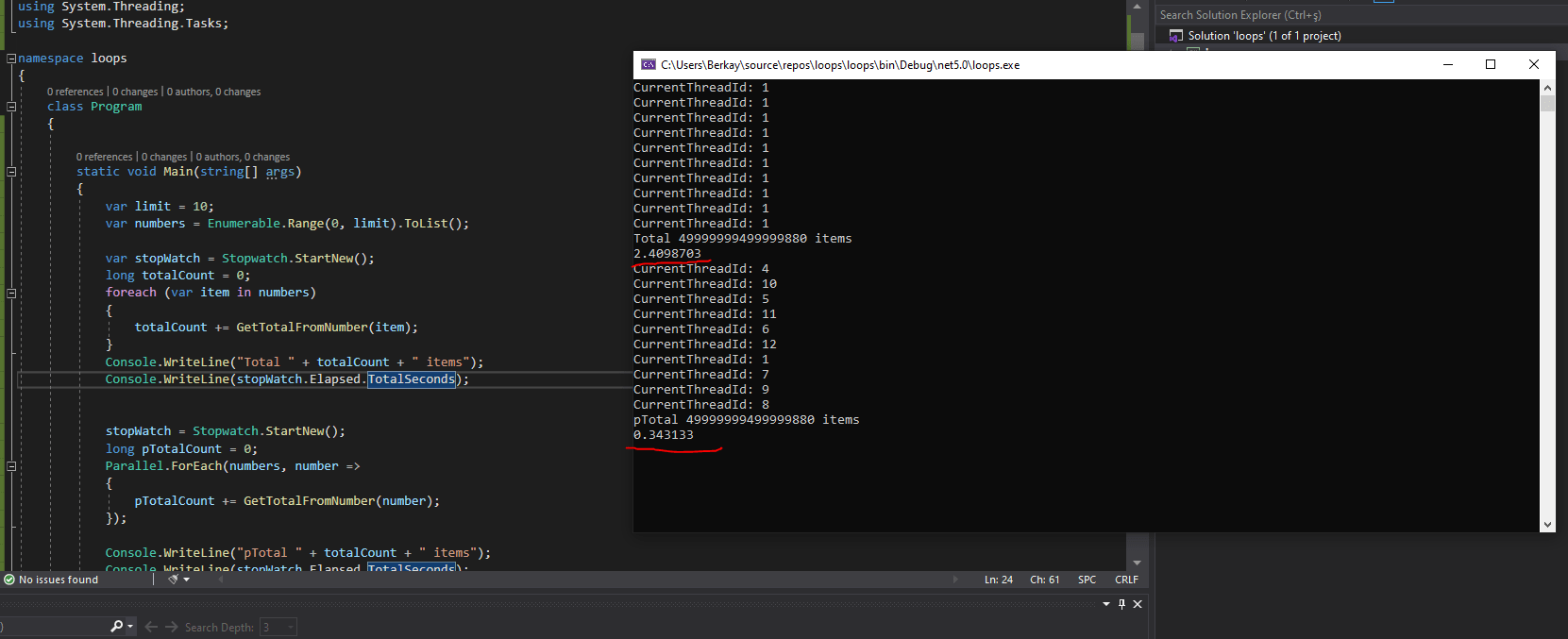Waow! 8 times faster then traditional foreach! But here is the thing as we mentioned before in this article. Parallel Foreach have used 10 different threads to achieve this operation. Traditional foreach used only single thread to do it.

In some reasons we don’t want our machine to consume all available threads like this. So we can limit degree of parallelism. Like below code

``````     var paralellism = new ParallelOptions
{
MaxDegreeOfParallelism = 3
};

Parallel.ForEach(numbers, paralellism, number =>
{
pTotalCount += GetTotalFromNumber(number);
});
``````

then considering above code, we will have output as below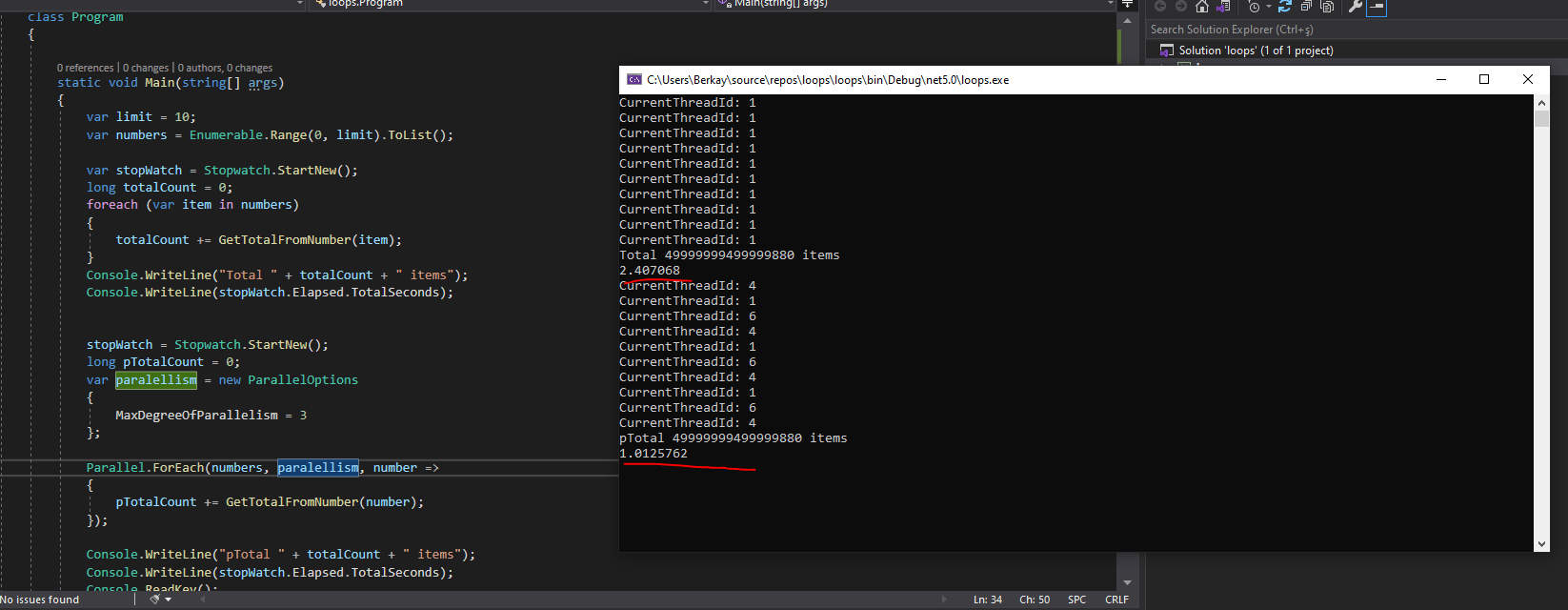We have limited thread usage with 3, but Parallel.Foreach() is still 2 times faster then traditional foreach().

You may also like to read:

Convert CSV to JSON in C#

Converting JSON to XML OR XML to JSON using C#

In Memory cache C# (Explanation with example in .NET and .NET Core)